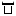```package com.example.demo.sf;

import java.util.ArrayList;

/**
* Created by caiqibin on 18/3/25.
*/
public class ChooseSoft {

public int[] crSoft(int [] arr){

for( int i = 1; i < arr.length-1; i++){
int temp = arr[i];//默认该位置最小
int j;
for(j = i ; j > 0 && arr[j-1] > temp; j--){
arr[j] =arr[j-1];
}
arr[j] = temp;
}
return arr;
}

public int [] xzSoft(int []arr){
for(int i = 0;i<arr.length-1;i++){
int min = i;
for(int j = i + 1; j<arr.length-1;j++){
if(arr[min] > arr[j]){
min = j;
}
if(min != i){
int temp = arr[min];
arr[min] = arr[i];
arr[i] = temp;
}
}
}
return arr;
}

public boolean testSoft(int [] arr){
for(int i=0;i<arr.length-1;i++){
if(arr[i] > arr[i+1]){
return false;
}
}
return true;
}

public  static void main(String args []){

int [] a={3,5,6,7,9,3,1,2,0,3};
ChooseSoft soft = new ChooseSoft();
int [] b = soft.crSoft(a);
for(int i = 0; i < b.length-1; i++) {
System.out.print(b[i]);
}
}
}
```最近下载更多
wohefeng456  LV1 2020年8月14日最近浏览更多
2022年5月18日

2022年5月18日扫描二维码关注最代码为好友